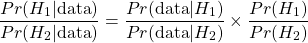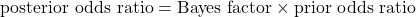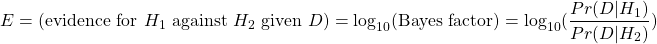# Quantifying Evidence (1): What Are Units of Evidence?

I am going to introduce a statistical framework for quantifying evidence as a series of blog posts. My hope is that by doing it through this format, people will understand it, build on these ideas, and actually use it as a practical replacement for p-value testing. If you haven’t already seen my post on why standard statistical methods that use p-values are flawed, you can check it out through this link.

My proposal builds on Bayesian hypothesis testing. Bayesian hypothesis testing makes use of the Bayes factor, which is the likelihood ratio of observing some data D for two competing hypotheses H1 and H2. A Bayes factor larger than 1 counts as evidence in favor of hypothesis H1; a smaller than one Bayes factor counts as evidence in favor of H2.

In classical hypothesis testing, we typically set a threshold for the p-value (say, p<0.01) below which a hypothesis can be rejected. But in the Bayesian framework, no such threshold can be defined as hypothesis rejection/confirmation will depend on the prior probabilities. Prior probabilities (i.e., the probabilities assigned prior to seeing data) are subjective. One person may assign equal probabilities for H1 and H2. Another may think H1 is ten times more likely than H2. And neither can be said to be objectively correct. But the Bayesian method leaves this subjective part out of the equation, allowing anyone to multiply the Bayes factor into their own prior probability ratio to obtain a posterior probability ratio. Depending on how likely you think the hypotheses are, you may require more or less evidence in order to reject one in favor of the other.Let us define ‘evidence‘ as the logarithm of the Bayes factor. The logarithmic scale is much more convenient to work with, as we will quickly see.Evidence is a quantity that depends on a particular observation or outcome and relates two hypothesis to one another. It can be positive or negative. For example, one can say Alice’s experimental results provide 3 units of evidence in favor of hypothesis H1 against hypothesis H2, or equivalently, -3 units of evidence in favor of hypothesis H2 against hypothesis H1.Figure 1: In this example, two hypotheses are being tested against one another. H1 is the hypothesis that the opossum is genetically closer to humpback whales than salmon is. H2 is the hypothesis that salmon is closer to humpback whales than the opossum. The data D that is being used to compare these hypotheses can be some DNA sequencing data, for instance. (The data here isn’t real).

But what does, for instance, 3 units of evidence mean? How do we interpret this number? 3 units of evidence means that it was 103=1000 times more likely to observe that particular outcome under hypothesis H1 compared to H2. And this number can be multiplied into one’s prior odds ratio to get a posterior odds ratio. If prior to seeing Alice’s data, you believed the probabiliy for H1 was half that of H2 (Pr(H1)/Pr(H2) = 0.5) then after seeing Alice’s data with 3 units of evidence, you update your probability odds ratio to Pr(H1)/Pr(H2) = 0.5×103 = 500. After seeing Alice’s data you attribute a probability to H1 that is 500 times larger than the probability you attribute to H2.

What’s nice about this definition is that evidence from independent observations can be added. This definition aligns with our colloquial usage of the term when we say “adding up” or “accumulating” evidence. So if Alice reports 3 units of evidence and Bob independently reports 2 units of evidence, it is as if we have a total of 5 units of evidence in favor H1 against H2. And if Carol then comes along with new experimental data providing 1.5 units of evidence in favor of H2 against H1 (conflicting with the other studies), the total resulting evidence is 3+2-1.5 = 3.5.

None of what I have written up to here is new. I am not even sure if my definition of evidence is entirely original. I’ve seen people use log likelihood ratios and call it evidence. But from here on is where we begin constructing something new.

It is commonly accepted that a scientific result needs to be replicated before it can be trusted. If two independent labs obtain congruent evidence for something (say Alice found 3 units of evidence and Bob found 2 units of evidence) it should count as stronger evidence than if just one of them found very strong evidence for it, (say Alice had instead found 5 units of evidence). But Bayes factors does not seem to reflect this very well since both cases are said to result in 5 units of evidence. To take this to an extreme, 10 independent studies all reporting 2 units of evidence in favor of H1 should prevail over one study reporting 20 units of evidence in favor of H2. But the way we currently set it up, they cancel each other out. How can we improve this framework to incorporate our intuition about the need for replication? I will discuss this in part 2.